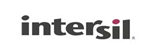# B180 PDF Datasheet浏览和下载B180
PDF下载：

ISL6844参考设计： ISL6844EVAL3Z [ISL6844 Reference Design: ISL6844EVAL3Z]

531 K

10 PagesINTERSIL [ INTERSIL CORPORATION ]Application Note 1612
ISL6844 Reference Design: ISL6844EVAL3Z
Introduction
This document focuses on Intersil’s solution for the flyback
converter. An inexpensive approach with discrete circuitry has
optimal performance are the prime objectives.
Intersil’s superior industry-standard ISL684x family of PWM
controllers would best serve the needs of this design. Some
key features of this family of parts include:
• 40ns peak current sensing
• 1A MOSFET driver
ISL6844 was selected for its large UVLO hysteresis, UVLO start
threshold, and the fact that the converter has been designed
for a maximum operational duty cycle of 50%, thus protecting
the IC by limiting the duty cycle in case of extreme fault
conditions.
Where:
η
= Converter’s efficiency, assuming 75%
P
OUT
= Total output power
F
sw
= Switching frequency
As a result, the peak magnitizing current = 1.06A
The transformer’s turn ratio can be determined from:
(
V
out
+
V
F
) ⋅ (
1
d
2
)
-
n
= -------------------------------------------------------
V
IN
,
MIN
d
MAX
d
MAX
+
d
2
<
1
(EQ. 2)
Where:
n = Turns ratio between the primary and the secondary
windings
V
F
= Forward drop across the diode, assuming 0.6V
d
2
= Duty cycle of diode conduction time
d
2
T
sw
is the magnitizing current reset time. Setting d
2
to 0.5,
Equation 2 yields the transformer’s turn ratio of 1.
D1
VIN
CIN
RS
DS
n
C
OUT2
D2
OUT
CS
VIN
RSENSE
VREG
VDD
n
-15V
CS
1
n
C
OUT1
+15V
Specifications
• Operating Input Voltage: 24V DC ±10%
• Output Voltage: ±15V
• Output Current: 100mA
• Ripple: 50mV
P-P
• Switching Frequency: 300kHz
• Topology: DCM Flyback
Design Procedure
Figure 1 shows a simplified circuit of the solution. It is
assumed that loads are balanced for both positive and
negative outputs. The turn ratio of the auxiliary winding is
chosen to be the same as the secondary winding. Figure 2
shows typical operational waveforms of a flyback converter in
discontinuous conduction mode.
ISL6844
FB
C
AUX
GND
Determine the Maximum Duty Cycle and
Transformer Turn Ratio
ISL6844 clamps the duty cycle to 50%. However, in this
converter design, it is assumed that the operating maximum
duty cycle, d
max
, will be 35% at the minimum input voltage of
21.6V.
Given the power level, the flyblack converter is designed to
operate in discontinuous conduction mode. The magnitizing
inductance can be calculated using Equation 1:
V
IN
,
MIN
d
max
-
L
M
=
η ⋅
----------------------------------------
2
P
out
F
sw
21.6
0.35
-
=
0.75
------------------------------------- =
23.8μH
3
2
3
300
×10
2
2
2
2
FIGURE 1. SIMPLIFIED CIRCUIT
(EQ. 1)
November 28, 2011
AN1612.1
1
CAUTION: These devices are sensitive to electrostatic discharge; follow proper IC Handling Procedures.
1-888-INTERSIL or 1-888-468-3774
|
Intersil (and design) is a registered trademark of Intersil Americas Inc.